# Basic Geometrical Ideas Notes

In this page we have Basic Geometrical Ideas Class 6 Notes. Hope you like them and do not forget to like , social share and comment at the end of the page.

## Point

• A point determines a location.
• It is usually denoted by a capital letter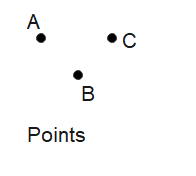## Line segment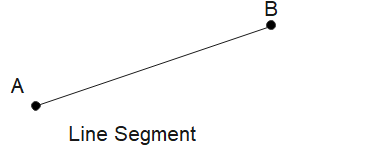• A line segment corresponds to the shortest distance between two points.
• The line segment joining points A and B is denoted by $\overline{AB}$.

## Line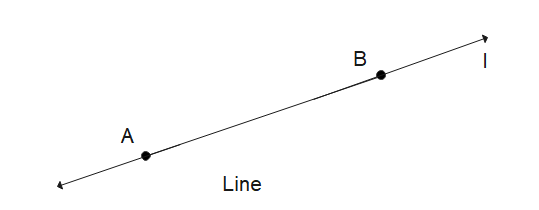• A line is obtained when a line segment like AB is extended on both sides indefinitely
• it is denoted by $\overleftrightarrow {AB}$ or sometimes by a single small letter like l.
• A line is a one-dimensional figure that is made up of an infinite number of points and extends infinitely in both directions. It is often denoted by the symbol l

## Ray• A ray is a portion of line starting at a point and going in one direction endlessly.
• It is represented as $\overrightarrow{AB}$, where
• A is the starting point and
• B is another point on the ray.

## Open Figures

An open figure is a shape that does not completely enclose a space. Examples include a line and a ray.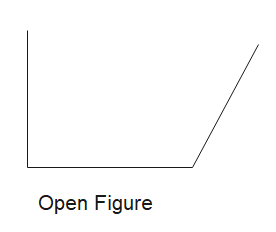## Closed Figures

A closed figure, on the other hand, completely encloses a space. Polygons like triangles, rectangles, and circles are examples of closed figures.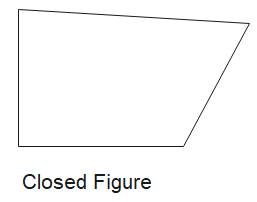## Interior and Exterior of Closed Figures

The interior of a closed figure refers to the area enclosed by the figure. The exterior is the area outside the closed figure but within the common boundary.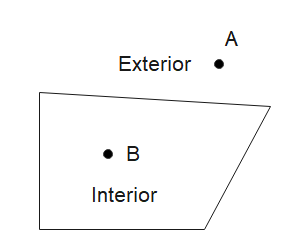## Curvilinear Boundaries

Curvilinear boundaries are boundaries formed by curved lines, like the boundary of a circle.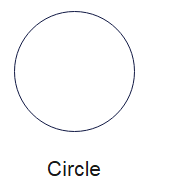## Linear Boundaries

Linear boundaries are boundaries formed by straight lines, as seen in polygons like triangles, rectangles, and squares.

## Angles,Vertex,Arm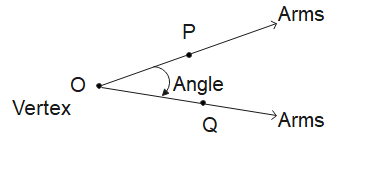• Angles:An angle is made up of two rays starting from a common initial point
• Vertex:The vertex of an angle is the point where two lines, rays, or line segments meet.
• Arm:The arms or the sidesare the two lines, rays, or line segments that form the angle.
• The angle name is given by the point on the sides and vertex.
• Here the angle is $\angle POQ$
• Note that in specifying the angle, the vertex is always written as the middle letter.

## Interior and Exterior of Angle

The interior of an angle is the space between the arms, and the exterior is the space outside the arms but within the angle's extensions.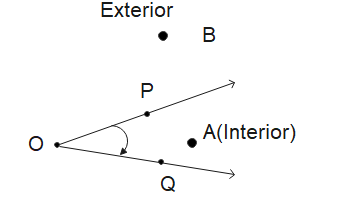## What is Polygon

• A polygon is simple closed figure made up entirely of line segments.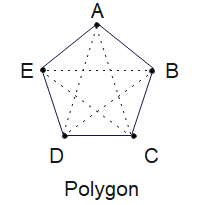• Sides: The line segments forming a polygon are called its sides.Here $\overline{AB}$,$\overline{BC}$,$\overline{CD}$, $\overline{DE}$, $\overline{EA}$
• Vertex : The meeting point of a pair of sides is called its vertex. A,B,C,D,E
• Adjacent sides: Any two sides with a common end point are called the adjacent sides of the polygon. Example $\overline{AB}$ & $\overline{BC}$ and $\overline{CD}$ & $\overline{DE}$
• Adjacent vertices:The end points of the same side of a polygon are called the adjacent vertices. Vertices A and B are Adjacent. Similarly B and C are Adjacent
• Diagonals: The joins of vertices which are not adjacent are called the diagonals of the polygon. AD, AC, EC,BD and BE are diagonals here

• Notes and NCERT Solutions
• Assignments### Practice Question

Question 1 What is $\frac {1}{2} + \frac {3}{4}$ ?
A)$\frac {5}{4}$
B)$\frac {1}{4}$
C)$1$
D)$\frac {4}{5}$
Question 2 Pinhole camera produces an ?
A)An erect and small image
B)an Inverted and small image
C)An inverted and enlarged image
D)None of the above